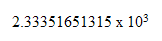# 2. Representing numbers

A CPU has a number of storage locations called 'registers'. These registers hold temporary data whilst processing is taking place. The registers hold binary bits that only have two values zero and one ( 0 or 1).

Therefore if a CPU is to process data, that data must be represented in binary form

A 'denary' number is one that is written using 'base 10' notation. For example 3.74, 14 orNumbers written like the one above are called 'scientific notation'

We use denary or base 10 numbering system every day and so we are very familiar with handling it. We know how to add, mulitply, divide and subtract denary numbers.

But you can represent a number in other 'bases'. For example 'base 8' (octal notation) 'base 16' (hexadecimal notation) and 'base 2' or 'binary notation'

Challenge see if you can find out one extra fact on this topic that we haven't already told you

Click on this link: number bases

I